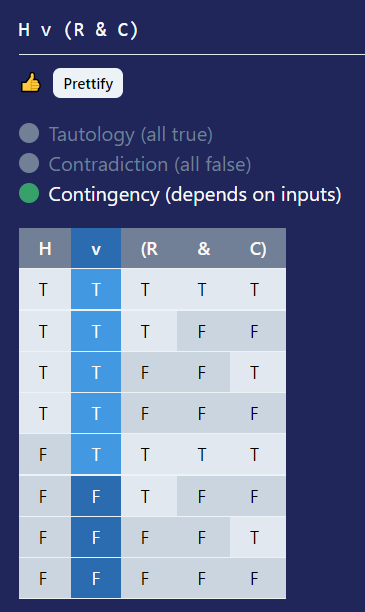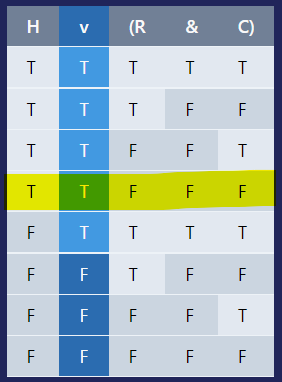TruthTables

Calculator

# The best truth table generator on the web

## Calculator

Generate a truth table for a symbolic logic statement.

Confused? Try

`~{[(A & G) v ~(U > ~S)] v I}`

## Logical operator quick reference

The first step in making a truth table is to translate your argument into the language of symbolic logic. Here's a logic translation cheat sheet to help out.

 Symbol Alternative Name Kind English connectives ~ ! Tilde Negation (NOT) it is not the case that A; not A; it is false that A & &&, /\ Ampersand Conjunction (AND) A and B; A but B; A yet B; A while B; A moreover B; A however B; A nonetheless B; A still B; A nevertheless B; A also B; A although B; both A and B; A additionally B; A furthermore B v ||, \/ Wedge Disjunction (OR) A or B; A unless B ⊃ > Horseshoe Conditional (IF) if A, then B; B only if A; A is a necessary condition for B; B is a necessary condition for A; B given that A; B provided that A; A implies that B; B on condition that A; B in case that A ≡ ==, = Triple bar Biconditional (IFF) A if and only if B; A just in case that B; A is a necessary and sufficient condition for B; A is equivalent to B

## What is a truth table?A truth table is a table that you can use to work with logic statements. Wikipedia says that:

A truth table is a mathematical table used in logic—specifically in connection with Boolean algebra, boolean functions, and propositional calculus—which sets out the functional values of logical expressions on each of their functional arguments, that is, for each combination of values taken by their logical variables. In particular, truth tables can be used to show whether a propositional expression is true for all legitimate input values, that is, logically valid.
But that's not very helpful if you're not already a logician. Basically, a truth table shows all of the possible inputs and outputs of a logic statement.

### What's a symbolic logic statement?

A symbolic logic statement is a way to represent a logical argument with symbols. So, you could write something like this:
I'm a human, or I'm a robot and I have a CPU for a brain.
into something like this:
H v (R & C)
To translate this into symbolic logic, we turned each of the atomic statements of the statement into single letter constants, and words like or and and into the corresponding logical operators (v and &). You can find a list of all logical operators here.

Now that we have a symbolic logic statement, we can generate a truth table for it. To do that, type it into the calculator. You should see an output like this:At the very top, there's a thumbs up emoji indicating the statement is well-formed. If you added an error into the statement (like changing "&" to "&&") that would go away and you'd see an error message. There's also a "Prettify" button to replace operators like > or = with nicer-looking versions. Next, you'll see indicators showing that the statement is a contingency. This simply means that the statement could either be true or false, depending on whether the inputs (the identifiers) are true or false. Statements can also be tautologies (the statement is always true, no matter what the inputs are) or contradictions (always false). For example, A v ~A is a tautology because if you translate it to English, it's saying that "A is true or it's false", which of course is always true regardless of what A is. A & ~A is a contradiction because it's saying that "A is true and it's false", and it's impossible for something to be both true and false at the same time. Our example is a contingency because it's possible for me to be neither a human or a robot, or I could be a robot but not have a CPU for a brain.

Finally, we have a truth table for our statement. Each component of our statement is broken out into a column of the table. The main operator's column is highlighted; this is the "output" of the statement. Let's try using it! First, we have to determine what the value of each identifier is. I'm a human, I'm not a robot, and I don't have a CPU for a brain, so H is true, R is false, and C is also false. On the table, we can find the row where each of our identifiers has the value we determined:And we can see that in this row, the main operator is true, meaning that the statement is true!

## How to use a truth table?To summarize, to make and use a truth table:

1. Translate your statement into symbolic logic
2. Paste your statement into the calculator to generate the truth table
3. Find the row on the table where the values of the identifiers match your input values
4. Read the value in the main operator column (highlighted) to see the result!

## FAQs### Are truth tables hard?

Truth tables can seem a bit intimidating at first, but once you get the hang of how to use them they're very straightforward. Using them is just a matter of plugging in values and finding the corresponding row.

### Why are truth tables useful?

Truth tables allow you to easily analyze a logical statement. It allows you to see the output of the statement for all combinations of inputs, which is helpful if the statement is very complicated and difficult to manually evaluate.

### What are some other terms for symbolic logic?

Symbolic logic can also be referred to as "formal logic," or more broadly, "mathematical logic." You can view more synonyms on Power Thesaurus (an excellent thesaurus site).